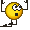# Rotational kinematic need explanation

• ice skater with mass = 80 kg
• moment of inertia (about her central axis) 3 kg m2.
• Catch baseball with outstretched arm 1m from her central axis.
• Ball has mass 0.3 kg and v0 = 20 m/s before the catch.
• V system ( skater + ball ) after catch = 0.0747 m/s
Question :
b. Angular speed of the system (skater + ball) after the catch ?
c. Percent Kinetic Energy lost during catch ?

Solution :
http://imgur.com/fj0Xstv
Is it correct ( answer for question c) ?

If yes, why K0 or kinetic energy before the catch doesn't have rotational kinetic energy ?
Ball has ω0 so it have rotational kinetic right ?

Related Introductory Physics Homework Help News on Phys.org
This solution assumes that V is the centre mass velocity after plastic collision. Angular momentum principle need because kinetic energy take not only for V, we have and rotation.

Angular momentum depends from the origin, so used only for the centre mass system.

This solution assumes that V is the centre mass velocity after plastic collision. Angular momentum principle need because kinetic energy take not only for V, we have and rotation.

Angular momentum depends from the origin, so used only for the centre mass system.
Thank you for your responese ,mihos.so is it correct the answer for question c ?

what is the answer for this one :
why K0 (in solution c) or kinetic energy before the catch doesn't have rotational kinetic energy ?
Ball has ω0 so it have rotational kinetic right ?

haruspex
Homework Helper
Gold Member
• ice skater with mass = 80 kg
• moment of inertia (about her central axis) 3 kg m2.
• Catch baseball with outstretched arm 1m from her central axis.
• Ball has mass 0.3 kg and v0 = 20 m/s before the catch.
• V system ( skater + ball ) after catch = 0.0747 m/s
Question :
b. Angular speed of the system (skater + ball) after the catch ?
c. Percent Kinetic Energy lost during catch ?

Solution :
http://imgur.com/fj0Xstv
Is it correct ( answer for question c) ?

If yes, why K0 or kinetic energy before the catch doesn't have rotational kinetic energy ?
Ball has ω0 so it have rotational kinetic right ?
I think the solution is not perfectly accurate because it treats the skater's mass centre as an inertial frame for the angular momentum calculation. I'll check later to see if that changes things. But let that pass for now.
You can view the ball has having linear KE or as having rotational KE about the skater's mass centre, but not as having both.

I think the solution is not perfectly accurate because it treats the skater's mass centre as an inertial frame for the angular momentum calculation. I'll check later to see if that changes things. But let that pass for now.
You can view the ball has having linear KE or as having rotational KE about the skater's mass centre, but not as having both.
Why not ? i'm confused.
How can ball that have both angular and linear speed only have 1 KE ?
Formula for KE = linear KE + rotational KE ? right ?
or is it because the ball is in the air so it can't roll (so no rotational movement)?

Thanks

haruspex
Homework Helper
Gold Member
Why not ? i'm confused.
How can ball that have both angular and linear speed only have 1 KE ?
Formula for KE = linear KE + rotational KE ? right ?
or is it because the ball is in the air so it can't roll (so no rotational movement)?

Thanks
You have no evidence that the ball is rotating on its own axis, and even if it were it would only be relevant if spinning on a vertical axis, and even then would have negligible result on the skater's rotation.

You have no evidence that the ball is rotating on its own axis, and even if it were it would only be relevant if spinning on a vertical axis, and even then would have negligible result on the skater's rotation.
Ball has ω0 = 20 rad/s , isn't that mean that the ball rotate ?
Also the ball has moment of inertia so that means it rotate on its central axis right ?haruspex